Home | | Maths 8th Std | Medians of a Triangle

# Medians of a Triangle

A median of a triangle is a line segment from a vertex to the midpoint of the side opposite that vertex.

Medians of a Triangle

A median of a triangle is a line segment from a vertex to the midpoint of the side opposite that vertex.

In the figureis a median of ∆ABC.Are there any more medians for ∆ABC ? Yes, since there are three vertices in a triangle, one can identify three medians in a triangle.

Example 5.16

In the figure, ABC is a triangle and AM is one of its medians. If BM = 3.5 cm, find the length of the side BC.Solution:

AM is median M is the midpoint of BC.

Given that, BM = 3.5 cm, hence BC = twice the length BM = 2 × 3.5 cm = 7 cm.

Activity

1. Consider a paper cut-out of a triangle. (Let us have an acute-angled triangle, to start with). Name it, say ABC.2. Fold the paper along the line that passes through the point A and meets the line BC such that point B falls on C. Make a crease and unfold the sheet.3. Mark the mid point M of BC.4. You can now draw the median AM, if  you want to see it clearly. (Or you can  leave it as a fold).5. In the same way, fold and draw the other two medians.6. Do the medians pass through the same point?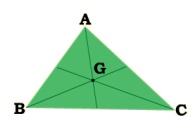Now you can repeat this activity for an obtuse - angled triangle and a right triangle.

What is the conclusion? We see that,

The three medians of any triangle are concurrent.

1. Centroid

The point of concurrence of the three medians in a triangle is called its Centroid, denoted by the letter G.Interestingly, it happens to be the centre of mass of the triangle. One can easily verify this fact. Take a sticut out of triangle of paper. It can be balanced horizontally at this point on a finger tip or a pencil tip.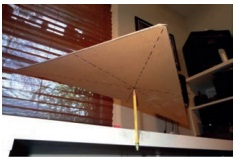Should you fold all the three medians to find the centroid? Now you can explore among yourself the following questions:

(i) How can you find the centroid of a triangle?

(ii)  Is the centroid equidistant from the vertices?

(iii)  Is the centroid of a triangle always in its interior?

(iv)  Is there anything special about the medians of an (a) Isosceles triangle? (b) Equilateral triangle?

Properties of the centroid of a triangle

The location of the centroid of a triangle exhibits some nice properties.

• It is always located inside the triangle.

• We have already seen that it serves as the Centre of gravity for any triangular lamina.

• Observe the figure given. The lines drawn from each vertex to G form the three triangles ∆ABG, ∆BCG, and ∆CAG.

Surprisingly, the areas of these triangles are equal.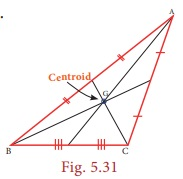That is, the medians of a triangle divide it into three smaller triangles of equal area!

The centroid of a triangle splits each of the medians in two segments, he one closer to the vertex being twice as long as the other one.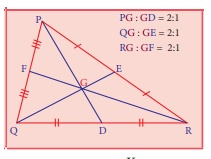This means the centroid divides each median in a ratio of 2:1. (For example, GD is ⅓ of PD).

(Try to verify this by paper folding).

Example 5.17

In the figure G is  the centroid of the triangle XYZ.

(i) If GL = 2.5 cm, find the length XL.

(ii) If YM = 9.3 cm, find the length GM.Solution:

(i) Since G is the centroid, XG : GL = 2 : 1 which gives XG : 2.5 = 2 : 1.

Therefore, we get 1 × (XG) = 2 × (2.5)

XG = 5 cm.

Hence, length XL = XG + GL = 5 + 2.5 = 7.5 cm.(ii) If YG is of 2 parts then GM will be 1 part. (Why?)

This means YM has 3 parts.

3 parts is 9.3 cm long. So GM (made of 1 part) must be 9.3 ÷ 3 = 3.1 cm.

Example 5.18

ABC is a triangle and G is its centroid. If AD=12 cm, BC=8 cm and BE=9 cm, find the perimeter of ∆BDG .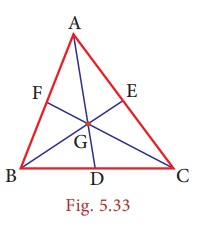Solution:

ABC is a triangle and G is its centroid. If,The perimeter of ∆BDG = BD+GD+BG = 4+4+6 = 14 cm

Solution 2:

ABC is a triangle and G is its centroid. If,

GD = 1/3 of AD = 1/3(12) = 4 cm and BE = 9 cm

BG = 2/3 of BE = 2/3 (9) = 6cm .

Also D is a midpoint of BC

BD = 1/2 of BC = 1/2 (8) = 4cm.

The perimeter of ∆BDG = BD+GD+BG = 4+4+6 = 14 cm

Tags : Geometry | Chapter 5 | 8th Maths , 8th Maths : Chapter 5 : Geometry
Study Material, Lecturing Notes, Assignment, Reference, Wiki description explanation, brief detail
8th Maths : Chapter 5 : Geometry : Medians of a Triangle | Geometry | Chapter 5 | 8th Maths